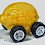## Thursday, September 3, 2015

### Price as a stochastic process

I mentioned the other day that I'd like to look into the Black Scholes equation in terms of information equilibrium. John Cochrane's Asset Pricing (2005) derives it using the asset pricing equation and a bit of stochastic calculus. I already looked at the basic asset pricing equation here, so I'm just going to set up the price in the information transfer framework as a stochastic process.

$$p \equiv \frac{dD}{dS} = \frac{1}{\kappa} \; \frac{D}{S}$$

So that

$$dp = \frac{1}{\kappa} \left( \frac{dD}{S} - \frac{D}{S^{2}} dS \right)$$

$$\frac{dp}{p} = \frac{dD}{D} - \frac{dS}{S}$$

Using the information equilibrium condition again, we have:

$$\frac{dp}{p} = \frac{dD}{D} - \kappa \frac{dD}{D}$$

$$\frac{dp}{p} = (1 - \kappa) \frac{dD}{D}$$

Let's say $D$ has a deterministic part and a stochastic part ...

$$\frac{dp}{p} = (1 - \kappa) \left( \frac{1}{D} \frac{\partial D}{\partial t} dt + \frac{1}{D} \frac{\partial D}{\partial x} dx \right)$$

Now if we identify

$$\mu \equiv (1 - \kappa) \frac{1}{D} \frac{\partial D}{\partial t}$$
$$\sigma \equiv (1 - \kappa) \frac{1}{D} \frac{\partial D}{\partial x}$$

We end up with

$$\frac{dp}{p} = \mu dt + \sigma dx$$

Which is a geometric stochastic process that would become the price $p$ of the underlying stock in the Black-Scholes option pricing model.

#### 1 comment:

1.